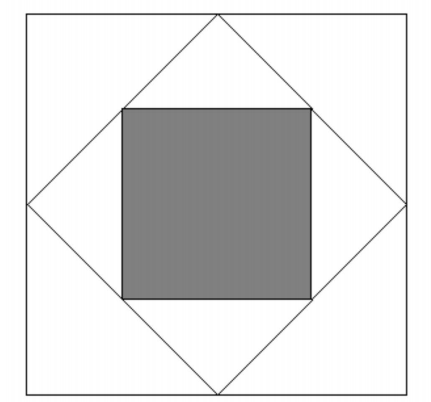Square Inception

Geometry Level 1The diagram above shows a large square, whose midpoints are connected up to form a smaller square. We then connect up the midpoints of the smaller square, to obtain the inner shaded square.

What fraction of the large square is shaded?

Note: Give your answer as a decimal to 2 decimal places.

×